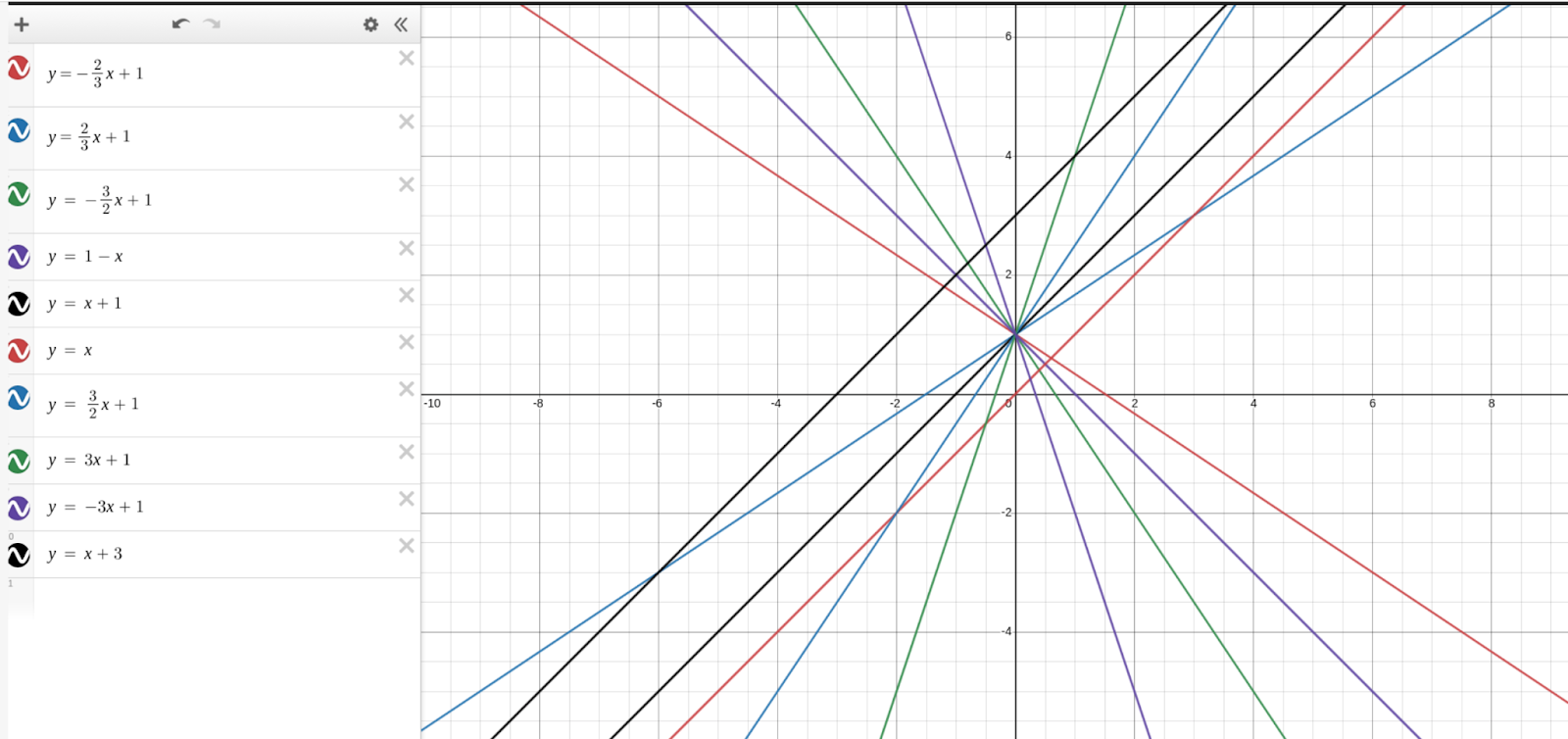# Multiple Choice vs. Free Response

When we give an assessment, it is our mission to understand the student who takes it as best we can. Multiple choice tests usually look to understand student performance, but we want to know how students think.

We did an experiment where we had the same students take the same question 3 years in a row. The first two years were multiple choice, the third year was a free-form answer. This is what we learned!

In the first two years, the options were:

A. y = x – 2/3

B. y = (-2/3)x + 1

C. y = (-3/2)x + 1

D. y = x – 3/2

In 2019, over 7,000 students took it and 81% chose option B, the correct answer.

In 2020, over 6,000 students in the same schools took it, and 78% chose option B.

For the students who chose option B in 2019, just over 70% chose it again in 2020.

When we asked it for the third time, in 2021, we took away multiple choice and had students write an equation. What do you think the results were? How many students got the right answer?

I’ll give you a little time to think about it.

Over 7,000 students took it and just over 40% got it right. That is half of what we were seeing in the 2 years of multiple choice. For the students who chose Option B in 2020, ~57% got the right equation in 2021. What happened?

It’s actually quite fascinating. 14% didn’t even write an equation but something else.

slope(m)=rise/run=-2/3

-3, 1, 3

But then, we also realized just how much more information is included in a free form answer rather than multiple choice.

You can really start to understand the students better. Are they writing equations when prompted? What form is that equation in? What did they write for the slope? What did they write for the y-intercept? All of these questions give you a picture of what students understand and what are they still working through.

Let’s take a deeper look!

Below is a graph of the top 10 equations students wrote:What do you notice?

We immediately noticed that students are writing equations with the correct intercept. They are all gravitating around that point. Let’s dive in.

Of the students who wrote an equation, ~83% of them got the right intercept. It is almost 90% for students who chose the right answer, Option B, in 2020. These students have a pretty good idea of how to find the intercept. If you go back to the multiple choice version, you’d see that students can get to a 50/50 chance, just from that.